# 1699 Sweet Candy Cane

### Today’s Puzzle:

Solving this candy cane puzzle can be a sweet experience. Just use logic to write the numbers 1 to 12 in both the first column and the top row so that those numbers and the given clues make a multiplication table.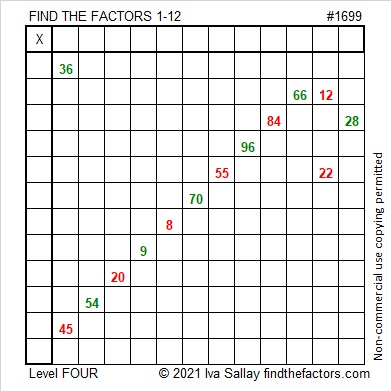### Factors of 1699:

• 1699 is a prime number.
• Prime factorization: 1699 is prime.
• 1699 has no exponents greater than 1 in its prime factorization, so √1699 cannot be simplified.
• The exponent in the prime factorization is 1. Adding one to that exponent we get (1 + 1) = 2. Therefore 1699 has exactly 2 factors.
• The factors of 1699 are outlined with their factor pair partners in the graphic below.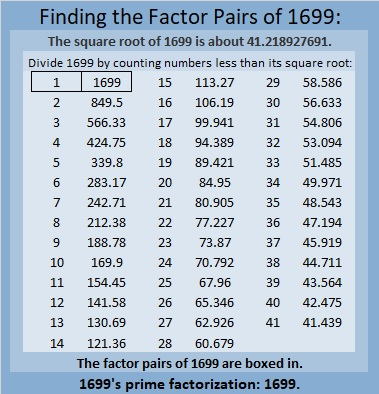How do we know that 1699 is a prime number? If 1699 were not a prime number, then it would be divisible by at least one prime number less than or equal to √1699. Since 1699 cannot be divided evenly by 2, 3, 5, 7, 11, 13, 17, 19, 23, 29, 31, 37, or 41, we know that 1699 is a prime number.

### More About the Number 1699:

1699 is the third prime in a prime triple. What are the other two primes in that triple?

1699 is the difference of two squares:
850² – 849² = 1699.

# 1687 Fly Me to the Moon!

### Today’s Puzzle:

A witch flying on a broomstick in front of a bright full moon is a common Halloween image. Here is a level 4 puzzle shaped like a broom. If you succeed in solving it, you might just feel like you are flying to the moon, too. Just write the numbers from 1 to 12 in both the first column and in the top row so that those numbers and the given clues form a multiplication table. Best Witches!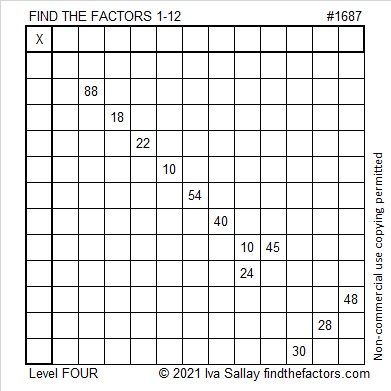### Factors of 1687:

1687 is divisible by 7 because 16 is the double of 8, and the last digit is 7.
217, 427, 637, 847, 1057, 1267, 1477, 1687, 1897 are all divisible by 7.

• 1687 is a composite number.
• Prime factorization: 1687 = 7 × 241.
• 1687 has no exponents greater than 1 in its prime factorization, so √1687 cannot be simplified.
• The exponents in the prime factorization are 1 and 1. Adding one to each exponent and multiplying we get (1 + 1)(1 + 1) = 2 × 2 = 4. Therefore 1687 has exactly 4 factors.
• The factors of 1687 are outlined with their factor pair partners in the graphic below.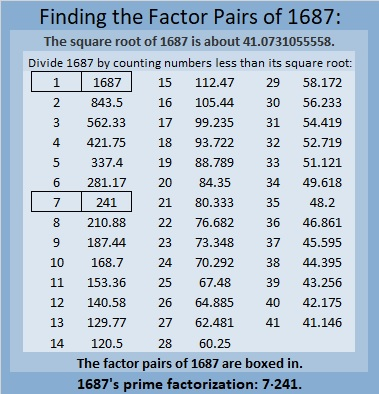### More About the Number 1687:

1687 is the hypotenuse of a Pythagorean triple:
840-1463-1687, which is 7 times (120-209-241).

# 1667 and Level 4

### Today’s Puzzle:

Use logic to write the numbers 1 to 12 in both the first column and the top row so that those numbers and the given clues function like a multiplication table.### Factors of 1667:

• 1667 is a prime number.
• Prime factorization: 1667 is prime.
• 1667 has no exponents greater than 1 in its prime factorization, so √1667 cannot be simplified.
• The exponent in the prime factorization is 1. Adding one to that exponent we get (1 + 1) = 2. Therefore 1667 has exactly 2 factors.
• The factors of 1667 are outlined with their factor pair partners in the graphic below.How do we know that 1667 is a prime number? If 1667 were not a prime number, then it would be divisible by at least one prime number less than or equal to √1667. Since 1667 cannot be divided evenly by 2, 3, 5, 7, 11, 13, 17, 19, 23, 29, 31, or 37, we know that 1667 is a prime number.

### More About the Number 1667:

Look at these consecutive number facts about the number 1667:
833 + 834 = 1667.
834² – 833² = 1667.

As the chart below shows, 1667 was ALMOST the fourth consecutive prime number ending in 7. Too bad prime number 1663 got in the way of that happening.# 1654 and Level 4

### Today’s Puzzle:

Use logic to write all the numbers 1 to 10 in both the first column and the top row of the puzzle so that those numbers are the factors of the given clues.### Factors of 1654:

• 1654 is a composite number.
• Prime factorization: 1654 = 2 × 827.
• 1654 has no exponents greater than 1 in its prime factorization, so √1654 cannot be simplified.
• The exponents in the prime factorization are 1 and 1. Adding one to each exponent and multiplying we get (1 + 1)(1 + 1) = 2 × 2 = 4. Therefore 1654 has exactly 4 factors.
• The factors of 1654 are outlined with their factor pair partners in the graphic below.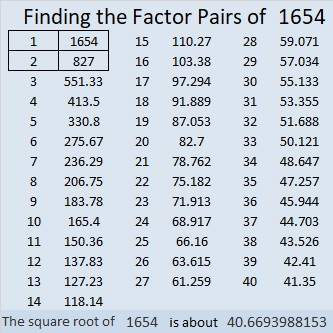### More About the Number 1654:

1654 is a leg in one Pythagorean triple:
1654-683928-683930, calculated from 2(827)(1), 827² – 1², 827² + 1².

# 1642 and Level 4

### Today’s Puzzle:

There are several clues in this puzzle with more than one factor pair, but if you use logic every step of the way, you can still write the factors 1 to 12 in the appropriate places to turn the puzzle into a multiplication table.### Factors of 1642:

• 1642 is a composite number.
• Prime factorization: 1642 = 2 × 821.
• 1642 has no exponents greater than 1 in its prime factorization, so √1642 cannot be simplified.
• The exponents in the prime factorization are 1 and 1. Adding one to each exponent and multiplying we get (1 + 1)(1 + 1) = 2 × 2 = 4. Therefore 1642 has exactly 4 factors.
• The factors of 1642 are outlined with their factor pair partners in the graphic below.### More About the Number 1642:

1642 is the sum of two squares:
39² + 11² = 1642.

1642 is the hypotenuse of a Pythagorean triple:
858-1400-1642, calculated from 2(39)(11), 39² – 11², 39² + 11².
That triple is also 2 times (429-700-821).

# 1631 The Importance of Practice

### Today’s Puzzle:

I did not have the privilege of learning a musical instrument when I was growing up, but I did make sure my children had that opportunity. One of the topics discussed in this next episode of Bill Davidson’s Podcast is the importance that practice plays in both music and mathematics. I thought it was quite good.

I think practicing is best when it is enjoyable. If you solve this musical note puzzle, it will hopefully be an enjoyable way for you to practice a few multiplication and division facts. Just use logic to write the numbers from 1 to 10 in both the first column and the top row so that those numbers and the given clues will function like a multiplication table.### Factors of 1631:

• 1631 is a composite number.
• Prime factorization: 1631 = 7 × 233.
• 1631 has no exponents greater than 1 in its prime factorization, so √1631 cannot be simplified.
• The exponents in the prime factorization are 1 and 1. Adding one to each exponent and multiplying we get (1 + 1)(1 + 1) = 2 × 2 = 4. Therefore 1631 has exactly 4 factors.
• The factors of 1631 are outlined with their factor pair partners in the graphic below.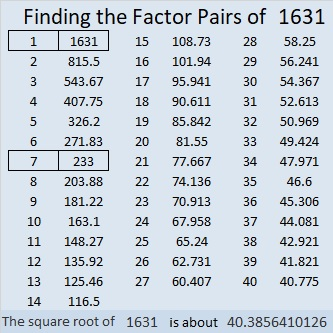### More about the Number 1631:

1631 is the hypotenuse of a Pythagorean triple:
735-1456-1631, which is 7 times (105-208-233).

1631 is the difference of two squares in two different ways:
816² – 815² = 1631, and
120² – 113² = 1631.

I found those number facts just from looking at the factors of 1631.

# 1598 See the Logic in This Level 4 Puzzle

### Today’s Puzzle:

Put the numbers from 1 to 10 in both the first column and the top row to turn this level 4 puzzle into a multiplication table. The logic in the ten clues is fairly straight-forward. Go ahead give it a try!### Factors of 1598:

• 1598 is a composite number.
• Prime factorization: 1598 = 2 × 17 × 47.
• 1598 has no exponents greater than 1 in its prime factorization, so √1598 cannot be simplified.
• The exponents in the prime factorization are 1, 1, and 1. Adding one to each exponent and multiplying we get (1 + 1)(1 + 1)(1 + 1) = 2 × 2 × 2 = 8. Therefore 1598 has exactly 8 factors.
• The factors of 1598 are outlined with their factor pair partners in the graphic below.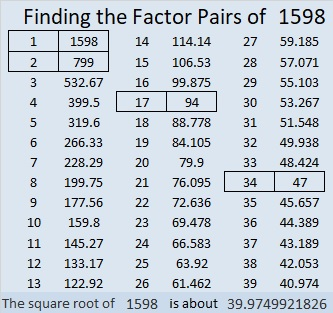### More about the Number 1598:

1598 is the hypotenuse of a Pythagorean triple:
752-1410-1598, which is (8-15-17) times 94.

# 1589 Candy Bars

### Today’s Puzzle:

Perhaps you can imagine that this level 4 puzzle looks like a couple of candy bars, one for you and one for me!### Factors of 1589:

Divisibility rules let us know quickly that 1589 is not divisible by 2, 3, or 5. Is it divisible by 7, the next prime number. We can apply the divisibility rule for 7 to find out:

1589 is divisible by 7 because
158 – 2(9) = 158 – 18 = 140, and 140 is divisible by 7.

• 1589 is a composite number.
• Prime factorization: 1589 = 7 × 227.
• 1589 has no exponents greater than 1 in its prime factorization, so √1589 cannot be simplified.
• The exponents in the prime factorization are 1 and 1. Adding one to each exponent and multiplying we get (1 + 1)(1 + 1) = 2 × 2 = 4. Therefore 1589 has exactly 4 factors.
• The factors of 1589 are outlined with their factor pair partners in the graphic below.### More about the Number 1589:

1589 is the difference of two squares two different ways:
795² – 794² = 1589, and
117² – 110² = 1589.

1589 is the sum of consecutive numbers in three different ways:
1589 is the sum of the two numbers from 794 to 795.
1589 is the sum of the seven numbers from 224 to 230.
1589 is also the sum of the fourteen numbers from 107 to 120,

# 1580 Use Logic to Solve This Puzzle

### Today’s Puzzle:

Where should you write the numbers from 1 to 10 in both the first column and the top row to make this puzzle function like a multiplication table? Study it until you find a logical place to start and continue to use logic until you’ve completed the puzzle.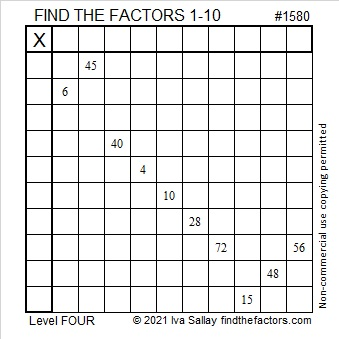### Factors of 1580:

• 1580 is a composite number.
• Prime factorization: 1580 = 2 × 2 × 5 × 79, which can be written 1580 = 2² × 5 × 79.
• 1580 has at least one exponent greater than 1 in its prime factorization so √1580 can be simplified. Taking the factor pair from the factor pair table below with the largest square number factor, we get √1580 = (√4)(√395) = 2√395.
• The exponents in the prime factorization are 2, 1, and 1. Adding one to each exponent and multiplying we get (2 + 1)(1 + 1)(1 + 1) = 3 × 2 × 2 = 12. Therefore 1580 has exactly 12 factors.
• The factors of 1580 are outlined with their factor pair partners in the graphic below.### More about the Number 1580:

1580 is the difference of two squares in two different ways:
396² – 394² = 1580, and
84² – 74² = 1580.

# 1562 Evergreen Tree

### Today’s Puzzle:

An evergreen tree doesn’t drop its leaves in the fall or look dead in the winter. As it reminds us of everlasting life, it makes a lovely symbol of Christmas.Write the numbers from 1 to 10 in both the first column and the top row so that those numbers and the given clues function like a multiplication table. Here is the same puzzle that won’t use as much ink to print:### Factors of 1562:

• 1562 is a composite number.
• Prime factorization: 1562 = 2 × 11 × 71.
• 1562 has no exponents greater than 1 in its prime factorization, so √1562 cannot be simplified.
• The exponents in the prime factorization are 1, 1, and 1. Adding one to each exponent and multiplying we get (1 + 1)(1 + 1)(1 + 1) = 2 × 2 × 2 = 8. Therefore 1562 has exactly 8 factors.
• The factors of 1562 are outlined with their factor pair partners in the graphic below.### Another Fact about the Number 1562:

2(5⁴ + 5³ + 5² + 5¹ + 5⁰) = 1562